International
Tables for
Crystallography
Volume C
Mathematical, physical and chemical tables
Edited by E. Prince

International Tables for Crystallography (2006). Vol. C, ch. 8.5, pp. 707-709
https://doi.org/10.1107/97809553602060000613

## Chapter 8.5. Detection and treatment of systematic error

E. Princea and C. H. Spiegelmanb

aNIST Center for Neutron Research, National Institute of Standards and Technology, Gaithersburg, MD 20899, USA, and bDepartment of Statistics, Texas A&M University, College Station, TX 77843, USA

Chapter 8.5 discusses the concept of accuracy and its difference from precision. Systematic error, or bias, results from an incorrect or incomplete model. Several ways to detect and eliminate bias, including measures of adequate fit, the effect of influential data, and plausibility of results, are discussed.

### 8.5.1. Accuracy

| top | pdf |

Chapter 8.4discusses statistical tests for goodness of fit between experimental observations and the predictions of a model with adjustable parameters whose values have been estimated by least squares or some similar procedure. In addition to the estimates of parameter values, one can also make estimates of the uncertainties in those values, estimates that are usually expressed in terms of an estimated standard deviation or, according to recommended usage (ISO, 1993), a standard uncertainty. A standard deviation is a measure of precision, that is, a measure of the width of a confidence interval that results from random fluctuations in the measurement process. What the experimenter who collected the data wants to know about, of course, is accuracy, a measure of the location of a region within which nature's `correct' value lies, as well as its width (Prince, 1994). In performing a refinement, we have assumed implicitly that the observations have been drawn at random from a population the mean of whose p.d.f. is given by a model when all of its parameters have those unknown, correct values. If this assumption is incorrect, the expected value of the estimate may no longer be near to the correct value, and the estimate contains bias, or systematic error. An accurate measurement is one that not only is precise but also has small bias. In this chapter, we shall discuss various criteria by which the results of a refinement may be judged in order to determine whether they are free of systematic error, and thus whether they may be considered accurate.

### 8.5.2. Lack of fit

| top | pdf |

We saw in Section 8.4.2that the sum of squared residuals from an ideally weighted, least-squares fit to a correct model is a sum of terms that has expected value (np) and is distributed as χ2 with ν = np degrees of freedom. Further, the residuals have a distribution with zero mean. A value for the sum that exceeds (np) by an amount that is improbably large is an indication of lack of fit, which may be due to an incorrect model for the mean or to nonideal weighting or both. {The sum, S, may be considered to be improbably large when the value of the χ2 cumulative distribution function,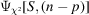, is close to 1.0. A value for the sum that is substantially less than (np) may also be an indication that the model contains more parameters than can be justified by the data set. Note also that a reasonable value for the sum of squared residuals does not prove that the model is correct. It indicates that the model adequately describes the data, but it in no way rules out the existence of alternative models that describe the data equally well.} If the sum of squares is greater than (np), it is commonly assumed that the mean model is correct, and that the weights have appropriate relative values, although their absolute values may be too large. If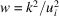, where k is some number greater than one, and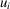is the standard uncertainty of the ith observation, the goodness-of-fit parameter,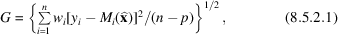is taken to be an estimate of k, and all elements of the inverse of the normal-equations matrix are multiplied by k2 to obtain the estimated variance–covariance matrix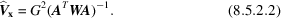Frequently, however, there is some other, independent estimate of the variance of the observation,, derived, for example, from counting statistics or from the observed scatter among symmetry-equivalent reflections. If this estimate is inconsistent with the hypothesis that all data points have been overweighted by a constant factor, then the assumption that the parameter estimates are unbiased but less precise than the original weights would indicate must be discarded. Instead, it must be assumed that the model is incorrect, or at least incomplete. A systematic error may be considered to cause the model to be incomplete, and may introduce bias into some or all of the refined parameters. (Note that in many standard statistical texts it is implicitly assumed, without so stating, that the data have already been scaled by a set of correct, relative weights. It is thus easy for the unwary reader to make the error of assuming that the practice of multiplying by the goodness-of-fit parameter is a well established procedure.)

The use of (8.5.2.2)to compute estimated variances and standard uncertainties assumes implicitly that the effect of lack of fit on parameter estimates is random, and applies equally to all parameters, even though different types of parameter may have very different mathematical relations in the model. With a model as complex as the crystallographic structure-factor formula, this assumption is certainly questionable.

Information about the nature of the model inadequacies can be obtained by examining the residuals (Belsley, Kuh & Welsch, 1980; Belsley, 1991). The standardized residuals,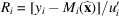, whereis the least-squares estimate of the parameters, should be randomly distributed, with zero mean, not only for the data set as a whole but also for subsets of the data that are chosen in a manner that depends only on the model and not on the observed values of the data. Here,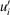is the standard uncertainty of the residual and is related to, the standard uncertainty of the observation, by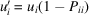, whereis a diagonal element of the projection matrix (Section 8.4.4). A scatter plot, in which the residuals are plotted against some control variable, such as |Fcalc|,, or one of the Miller indices, should reveal no general trends. The existence of any such trend may indicate a systematic effect that depends on the corresponding variable. The model may then be modified by inclusion of a factor that is proportional to that variable, and the refinement repeated. An examination of the shifts in the other parameters, and of the new row or column of the variance–covariance matrix, will then reveal which of the parameters in the unmodified model are likely to have been biased by the systematic effect. When this procedure has been followed, it is extremely important to consider carefully the nature of the additional effect and determine whether it is plausible in terms of physics and chemistry.

Another procedure for detecting systematic lack of fit makes use of the fact that, if the model is correct, and the error distribution is approximately normal, or Gaussian, the distribution of residuals will also be approximately normal. A large sample may be checked for normality by means of a quantile–quantile, or QQ, plot (Abrahams & Keve, 1971; Kafadar & Spiegelman, 1986). To make such a plot, the residuals are first sorted in ascending order of magnitude. If there are n points in the data set, the value of the ith sorted residual should be close to the value, xi, for which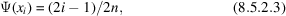where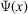is the cumulative distribution function for the normal p.d.f. A plot of Ri against xi should be a straight line with zero intercept and unit slope. A straight line with a slope greater than one suggests that the model is satisfactory, but that the variances of the data points have been systematically underestimated. Lack of fit is suggested if the curve has a higher slope near the ends, indicating that large residuals occur with greater frequency than would be predicted by the normal p.d.f.

The sorted residuals tend to be strongly correlated. A positive displacement from a smooth curve tends to be followed by another positive displacement, and a negative one by another negative one, which gives the QQ plot a wavy appearance, and it may be difficult to decide whether it is a straight line or not. Because of this, a useful alternative to the QQ plot is the conditional QQ plot (Kafadar & Spiegelman, 1986), so called because the abscissa for plotting the ith sorted residual is the mean of a conditional p.d.f. for that residual given the observed values of all the others. To construct a conditional QQ plot, first transform the distribution to a uniform p.d.f. by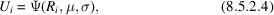where μ and σ are resistant estimates (Section 8.2.2) of the mean and standard deviation of the p.d.f., such as the median and 0.75 times the interquartile range, and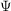represents the cumulative distribution function. Letting U0 = 0 and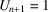, the expected value of Ui, given all the others, is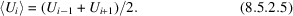The ith abscissa for the QQ plot is then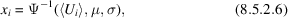where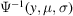is a per cent point function, or p.p.f., the value of x for which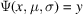.

QQ plots for subsets of the data can reveal, by nonzero intercepts, that those subsets are subject to a systematic bias. Because of its property of removing short-range kinks in the curve, the conditional QQ plot can be particularly useful in this application. The values of μ and σ used for the transformation to a uniform distribution, as in (8.5.2.4), should be those determined from the entire data set.

A QQ plot will reveal data points that are in poor agreement with the model, but that do not belong to any easily identifiable subset. Because of the central limit theorem (Section 8.4.1), however, the least-squares method tends to force the distribution of the residuals toward a normal distribution, and the discrepant points may not be clearly evident. A robust/resistant procedure (see Section 8.2.2), because it reduces the influence of strongly discrepant data points, helps to separate them from the body of the data. Therefore, if a data set contains discrepant points, a QQ plot of the residuals from a robust/resistant fit will tend to have greater curvature at the extremes than one from a corresponding least-squares fit. If the discrepant data points that are thus identified have a pattern, this information may enable a systematic error to be characterized.

### 8.5.3. Influential data points

| top | pdf |

Section 8.4.4discusses the influence of individual data points on the estimation of parameters and how to identify the data points that should be measured with particular care in order to make the most precise estimates of particular parameters. The same properties that cause these influential data points to be most effective in reducing the uncertainty of a parameter estimate when the model is a correct predictor for the observations also cause them to have the greatest potential for introducing bias if there is a flaw in the model or, correspondingly, if they are subject to systematic error. Reviews of procedures for studying the effects of influential data points and outliers have been given by Beckman & Cook (1983), by Chatterjee & Hadi (1986), and by Belsley (1991).

The effects of possible systematic error can be studied by identifying influential data points and then observing the effects of deleting them one by one from the refinement. The deletion of a data point should affect the standard uncertainty of an estimate, but should not cause a shift in its mean that is more than a small multiple of the resulting standard uncertainty. As in Section 8.4.4, we define the design matrix, A, by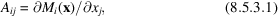whereis the model function for the ith data point, and x is a vector of adjustable parameters. Let R be the upper triangular Cholesky factor of the weight matrix, so that W = RTR, and define the weighted design matrix by Z = RA and the weighted vector of observations by y′ = Ry. The least-squares estimate of x is thenand the vector of predicted values is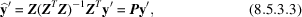where P is the projection, or hat, matrix. A diagonal element,, of P is a measure of the leverage, that is of the relative influence, of the ith data point, and therefore of the sensitivity of the estimates of the elements of x to an error in the measurement of that data point.lies in the range, and it has average value p/n, so that data points with values ofgreater than 2p/n can be considered particularly influential.

Let H = ZTZ be the normal-equations matrix, let V = H−1 be the estimated variance–covariance matrix, and let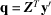, so that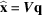. Let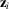be the ith row of Z, and denote by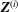,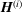,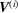,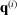, and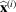the respective matrices and vectors computed with the ith data point deleted from the data set. We wish to find large values of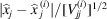, so we need to computeand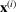. With a derivation similar to that for (8.4.4.7), it can be shown (Fedorov, 1972; Prince & Nicholson, 1985) that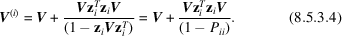Note that, if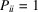, all elements ofbecome infinite, implying thatis singular. Thus, if such a data point is deleted, the solution is no longer determinate. Now,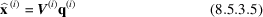and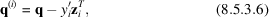so that, when V and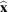have been computed once, it is a straightforward and inexpensive additional computation to determine whether any parameter has been strongly influenced, and therefore potentially biased, by the inclusion of any data point in the refinement. If there is any reason to be concerned about possible systematic error, the leverage of every data point included in the refinement should be computed, and the effects of deletion of all of those with leverage greater than 2p/n should be observed.

### 8.5.4. Plausibility of results

| top | pdf |

A study of residuals to detect a pattern of discrepancies will reveal the presence of systematic error, or model inadequacy, only if different subsets of the data are affected differently. Some sources of bias, however, have noticeable effects throughout the data set, and missing parameters may mimic others that have been included, thus introducing bias without any apparent lack of fit. To cite an obvious example, determination of unit-cell dimensions requires an accurate value of the wavelength of the radiation being used. If this value is incorrect, inferred values of the cell constants may be reproduced repeatedly with great precision, but all will be subject to a systematic bias that has no effect on the quality of the fit. Even though the structure of the residuals in such a case reveals little about possible systematic error, it is still possible to detect it by critical examination of the estimated parameters.

Even before any data have been collected in preparation for the determination of a crystal structure, a great deal is known about certain details. It is known that the crystal is composed of atoms of certain elements that are present in certain proportions. It is known that pairs of atoms of various elements cannot be less than a certain distance apart, and, further, that adjacent atoms tend to be separated by distances that fall within a rather narrow range. It is known that thermal vibration amplitudes are likely to be larger in directions normal to interatomic vectors than parallel to them, although, particularly in the case of hydrogen bonds, there may be an apparent amplitude parallel to a vector because of atomic disorder. Even when there is a particularly unusual feature in a structure, most of the structure will conform to commonly observed patterns. Thus, if a refined crystal structure overall has reasonable features, such as interatomic distances that are appropriate to oxidation state and coordination number and displacement ellipsoids that make sense, one or two unusual features may be accepted with confidence. On the other hand, if there is wide variation in the lengths of chemically similar bonds, or if the eigenvectors of the thermal motion tensors point in odd directions relative to the interatomic vectors, there must be a presumption that systematic errors have been compensated for by biased estimates of parameters.

A particular problem arises when there is a question of the presence or absence of symmetry, such as a choice between two space groups, one of which possesses a centre of symmetry or a mirror plane, or a case where a symmetric molecule occupies a position whose environment has a less-symmetric point group. If symmetry constraints are relaxed, the model can always be refined to a lower sum of squared residuals. (For a discussion of numerical problems that occur in the vicinity of a symmetric configuration, see Section 8.1.3.) The problem comes from the fact that the removal of the symmetry element almost always introduces too many additional parameters. Statistical tests are then quite likely to indicate that the lower-symmetry model gives a significantly better fit, but consideration of internal consistency and chemical or physical plausibility is likely to suggest that much systematic error has been absorbed by the additional parameters. The proper procedure is to devise a model with noncrystallographic constraints (see Section 8.3.2) that expresses what is, for chemical or physical reasons, known or probable. To do so may require great patience and perseverance.

### References

Abrahams, S. C. & Keve, E. T. (1971). Normal probability plot analysis of error in measured and derived quantities and standard deviations. Acta Cryst. A27, 157–165.
Beckman, R. J. & Cook, R. D. (1983). Outlier..........s. Technometrics, 25, 119–149.
Belsley, D. A. (1991). Conditioning diagnostics. New York: John Wiley & Sons.
Belsley, D. A., Kuh, E. & Welsch, R. E. (1980). Regression diagnostics. New York: John Wiley & Sons.
Chatterjee, S. & Hadi, A. S. (1986). Influential observations, high leverage points, and outliers in linear regression. Stat. Sci. 1, 379–393.
Fedorov, V. V. (1972). Theory of optimal experiments, translated by W. J. Studden & E. M. Klimko. New York: Academic Press.
ISO (1993). Guide to the expression of uncertainty in measurement. Geneva: International Organization for Standardization.
Kafadar, K. & Spiegelman, C. H. (1986). An alternative to ordinary Q–Q plots: conditional Q–Q plots. Comput. Stat. Data Anal. 4, 167–184.
Prince, E. (1994). Mathematical techniques in crystallography and materials science, 2nd ed. Berlin/Heidelberg/New York/London/Paris/Tokyo/Hong Kong/Barcelona/Budapest: Springer-Verlag.
Prince, E. & Nicholson, W. L. (1985). Influence of individual reflections on the precision of parameter estimates in least squares refinement. Structure and statistics in crystallography, edited by A. J. C. Wilson, pp. 183–195. Guilderland, NY: Adenine Press.